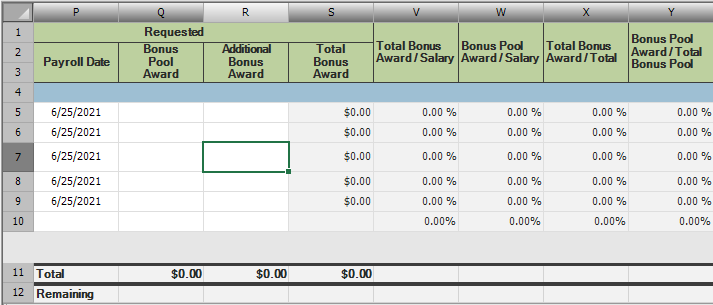SheetViewCellChanged row is incorrect when cell is referenced by a formula cell

Posted by: TBorek on 15 April 2021, 6:59 am EST

• Post Options:

Posted 15 April 2021, 6:59 am EST

When setting values in the spreadsheet, the SheetViewCellChanged event includes the wrong row index in the SheetViewEventArgs.
No matter which cell in the range (Q5:R9) I try to fill in, the event args e.Row always returns 4, no matter which row it actually is. e.Column is the correct value.

This appears to be related to the formulas in column X and Y. If I comment out just the lines where the formulas are set and run it again, the SheetViewCellChanged event passes in the correct row index, (i.e., updating cell R7 passes in e.Row=6)

If only column X exists then filling in data with SheetViewEventArgs is broken for both column Q and R.
If only column Y exists then filling in data with SheetViewEventArgs is broken for only column Q.
Filling in data for other columns cells works perfectly. It appears to be directly related to the cells the formulas in columns X and Y are referencing, directly or indirectly.

The values for all the formula cells evaluate to the correct values, it is only SheetViewCellChanged.SheetViewEventArgs that is passing in incorrect data.

Filling in cell Q7 results in the following SheetViewEventArgs values:
e.Column = 16
e.ColumnCount = 15
e.Row = 4
e.RowCount = 7

After commenting out the formula for the offending columns (X,Y) the SheetViewEventArgs contains the following values for cell Q7:
e.Column = 16
e.ColumnCount = 15
e.Row = 6
e.RowCount = 5

I have attempted to change the formula to not reference any calculated cells, same issue. I have tried setting SheetView.AutoCalculation = False and calling SheetView.Recalculate() manually, same issue. I have tried moving where I assign the formula to the cells, same issue.

Below is the layout of the spreadsheet, some irrelevant columns are hidden:Below is the SheetViewCellChanged function:

Private Sub SheetViewCellChanged(ByVal sender As Object, ByVal e As SheetViewEventArgs)

'We don't want our changes we make here to call this method
_isInCellChanged = True

Try
Dim currentRow As Integer = e.Row ' THIS VALUE IS WRONG
Dim currentCol As Integer = e.Column
Dim selectedCell = sheetView.Cells(currentRow, currentCol)

If selectedCell IsNot Nothing Then
'Update Data
End If

Finally
_isInCellChanged = False
End Try
End Sub

Finally the formulas for the different cells:

Data Cells:

Total Bonus Award:
SheetView.Cells(S5).Formula = SUM(Q5:R5)
SheetView.Cells(S6).Formula = SUM(Q6:R6)
SheetView.Cells(S7).Formula = SUM(Q7:R7)
...

Totals:

Total Bonus Award Total Cell:
SheetView.Cells(S11).Formula = SUBTOTAL(9,S5:S10)

Bonus Pool Award Total Cell:
SheetView.Cells(Q11).Formula = SUBTOTAL(9,Q5:Q10)

SheetView.Cells(R11).Formula = SUBTOTAL(9,R5:R10)

Percentage Cells:

Total Bonus Award / Salary - These work fine:
SheetView.Cells(V5).Formula = IF(N5=0, 0, S5/N5)
SheetView.Cells(V6).Formula = IF(N6=0, 0, S6/N6)
SheetView.Cells(V7).Formula = IF(N7=0, 0, S7/N7)

Bonus Pool Award / Salary - These work fine:
SheetView.Cells(W5).Formula = IF(N5=0, 0, Q5/N5)
SheetView.Cells(W6).Formula = IF(N6=0, 0, Q6/N6)
SheetView.Cells(W7).Formula = IF(N7=0, 0, Q7/N7)

Total Bonus Award / Total - These cause issues:
SheetView.Cells(X5).Formula = IF(S11=0, 0, S5/S11)
SheetView.Cells(X6).Formula = IF(S11=0, 0, S6/S11)
SheetView.Cells(X7).Formula = IF(S11=0, 0, S7/S11)

Bonus Pool Award / Total Bonus Pool - These cause issues:
SheetView.Cells(Y5).Formula = IF(Q11=0, 0, Q5/Q11)
SheetView.Cells(Y6).Formula = IF(Q11=0, 0, Q6/Q11)
SheetView.Cells(Y7).Formula = IF(Q11=0, 0, Q7/Q11)
• Post Options:

Replied 16 April 2021, 12:28 am EST

Hi,

Thanks for the detailed info on this issue. I was able to replicate this at my end and I've asked the developers to take a look at this [SPNET-16862].

I'll update this thread once we get any information for you.

Regards,
Jitender

• Post Options:

Replied 18 April 2021, 3:19 pm EST

Hi,

This is the default behavior of Spread. The CellChanged event is fired for a CellRange.
If you want it to fire for each Cell, you can use the UnionedChangeCellRange property:

Regards,
Jitender
Need extra support?

Upgrade your support plan and get personal unlimited phone support with our customer engagement team

• ComponentOne

Forums for all current editions of the ComponentOne .NET UI control product line, including ComponentOne Studio and ComponentOne Studio for Xamarin.

• ActiveReports

Forums for all versions of ActiveReports and ActiveReports Server